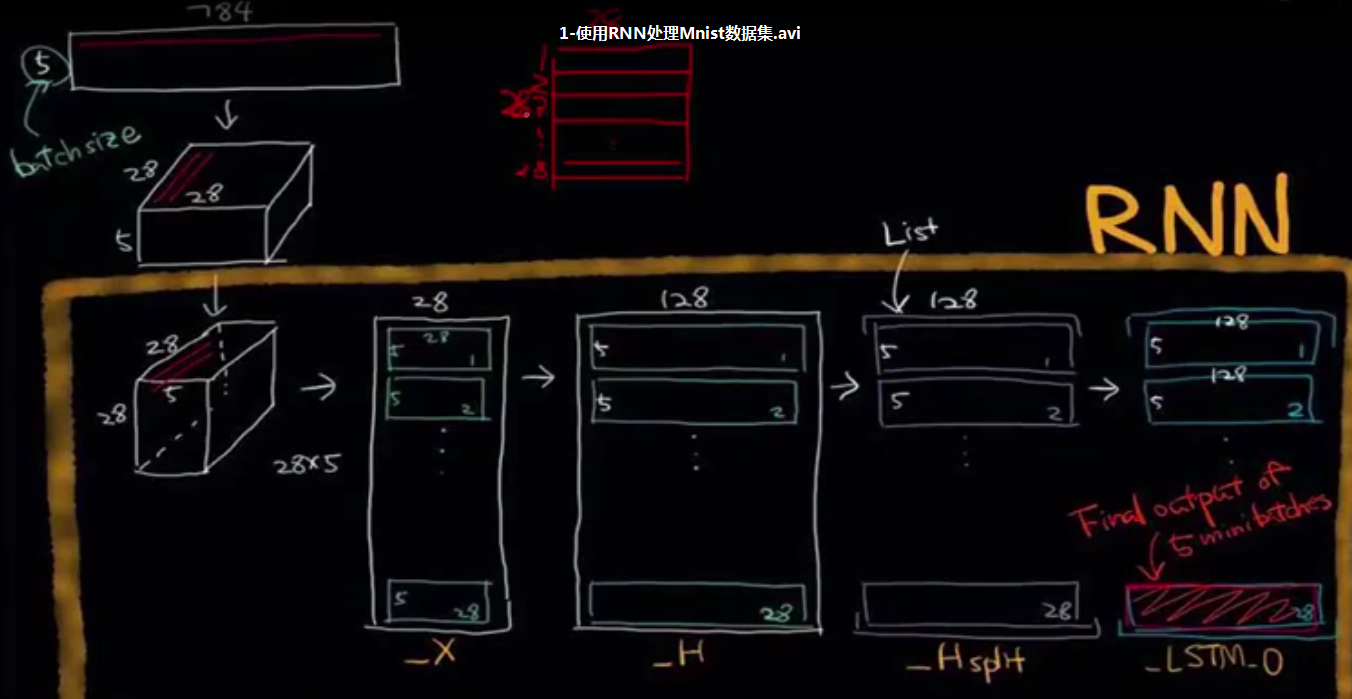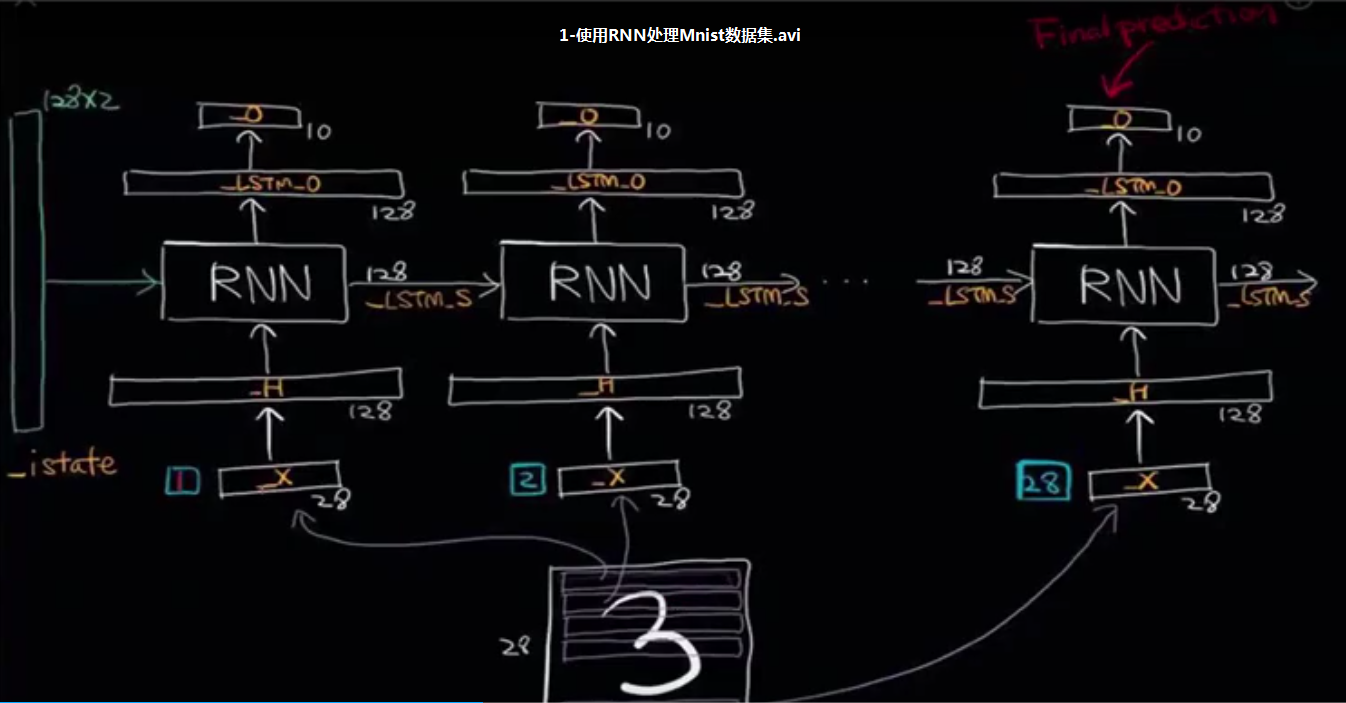# tensorflow学习5-RNN网络

### 1.原理图:### 2.代码:

import numpy as np
import tensorflow as tf
import tensorflow.examples.tutorials.mnist.input_data as input_data

trainimgs, trainlabels, testimgs, testlabels \
= mnist.train.images, mnist.train.labels, mnist.test.images, mnist.test.labels
ntrain, ntest, dim, nclasses \
= trainimgs.shape, testimgs.shape, trainimgs.shape, trainlabels.shape

diminput  = 28
dimhidden = 128
dimoutput = nclasses
nsteps    = 28
weights = {
'hidden': tf.Variable(tf.random_normal([diminput, dimhidden])),
'out': tf.Variable(tf.random_normal([dimhidden, dimoutput]))
}
biases = {
'hidden': tf.Variable(tf.random_normal([dimhidden])),
'out': tf.Variable(tf.random_normal([dimoutput]))
}

def _RNN(_X, _W, _b, _nsteps, _name):
# 1. Permute input from [batchsize, nsteps, diminput]
#   => [nsteps, batchsize, diminput]
_X = tf.transpose(_X, [1, 0, 2])
# 2. Reshape input to [nsteps*batchsize, diminput]
_X = tf.reshape(_X, [-1, diminput])
# 3. Input layer => Hidden layer
_H = tf.matmul(_X, _W['hidden']) + _b['hidden']
# 4. Splite data to 'nsteps' chunks. An i-th chunck indicates i-th batch data
_Hsplit = tf.split(_H, _nsteps,0 )
# 5. Get LSTM's final output (_LSTM_O) and state (_LSTM_S)
#    Both _LSTM_O and _LSTM_S consist of 'batchsize' elements
#    Only _LSTM_O will be used to predict the output.
with tf.variable_scope(_name) as scope:
#scope.reuse_variables()
lstm_cell = tf.nn.rnn_cell.BasicLSTMCell(dimhidden, forget_bias=1.0)
_LSTM_O, _LSTM_S = tf.nn.static_rnn(lstm_cell, _Hsplit, dtype=tf.float32)
# 6. Output
_O = tf.matmul(_LSTM_O[-1], _W['out']) + _b['out']
# Return!
return {
'X': _X, 'H': _H, 'Hsplit': _Hsplit,
'LSTM_O': _LSTM_O, 'LSTM_S': _LSTM_S, 'O': _O
}

learning_rate = 0.001
x      = tf.placeholder("float", [None, nsteps, diminput])
y      = tf.placeholder("float", [None, dimoutput])
myrnn  = _RNN(x, weights, biases, nsteps, 'basic')
pred   = myrnn['O']
cost   = tf.reduce_mean(tf.nn.softmax_cross_entropy_with_logits(logits=pred, labels=y))
accr   = tf.reduce_mean(tf.cast(tf.equal(tf.argmax(pred,1), tf.argmax(y,1)), tf.float32))
init   = tf.global_variables_initializer()

training_epochs = 5
batch_size      = 16
display_step    = 1
sess = tf.Session()
sess.run(init)
print ("Start optimization")
for epoch in range(training_epochs):
avg_cost = 0.
#total_batch = int(mnist.train.num_examples/batch_size)
total_batch = 100
# Loop over all batches
for i in range(total_batch):
batch_xs, batch_ys = mnist.train.next_batch(batch_size)
batch_xs = batch_xs.reshape((batch_size, nsteps, diminput))
# Fit training using batch data
feeds = {x: batch_xs, y: batch_ys}
sess.run(optm, feed_dict=feeds)
# Compute average loss
avg_cost += sess.run(cost, feed_dict=feeds)/total_batch
# Display logs per epoch step
if epoch % display_step == 0:
print ("Epoch: %03d/%03d cost: %.9f" % (epoch, training_epochs, avg_cost))
feeds = {x: batch_xs, y: batch_ys}
train_acc = sess.run(accr, feed_dict=feeds)
print (" Training accuracy: %.3f" % (train_acc))
testimgs = testimgs.reshape((ntest, nsteps, diminput))
feeds = {x: testimgs, y: testlabels}
test_acc = sess.run(accr, feed_dict=feeds)
print (" Test accuracy: %.3f" % (test_acc))
print ("Optimization Finished.")


### 3.结果:

Network Ready!
2019-05-17 14:17:19.454400: I tensorflow/core/platform/cpu_feature_guard.cc:141] Your CPU supports instructions that this TensorFlow binary was not compiled to use: AVX2
Start optimization
Epoch: 000/005 cost: 1.681037109
Training accuracy: 0.625
2019-05-17 14:17:24.844400: W tensorflow/core/framework/allocator.cc:124] Allocation of 143360000 exceeds 10% of system memory.
Test accuracy: 0.440
Epoch: 001/005 cost: 1.285484257
Training accuracy: 0.750
2019-05-17 14:17:31.432400: W tensorflow/core/framework/allocator.cc:124] Allocation of 143360000 exceeds 10% of system memory.
Test accuracy: 0.565
Epoch: 002/005 cost: 1.131057074
Training accuracy: 0.625
2019-05-17 14:17:37.846400: W tensorflow/core/framework/allocator.cc:124] Allocation of 143360000 exceeds 10% of system memory.
Test accuracy: 0.592
Epoch: 003/005 cost: 0.991629310
Training accuracy: 0.688
2019-05-17 14:17:44.179400: W tensorflow/core/framework/allocator.cc:124] Allocation of 143360000 exceeds 10% of system memory.
Test accuracy: 0.614
Epoch: 004/005 cost: 0.931213274
Training accuracy: 0.812
2019-05-17 14:17:50.471400: W tensorflow/core/framework/allocator.cc:124] Allocation of 143360000 exceeds 10% of system memory.
Test accuracy: 0.593
Optimization Finished.©️2019 CSDN 皮肤主题: 终极编程指南 设计师: CSDN官方博客